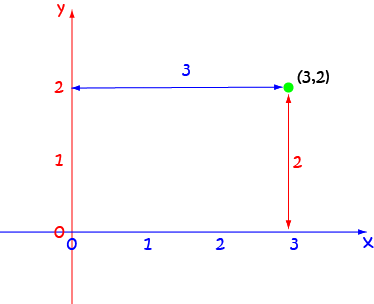# Definition of Ordered PairAn ordered pair consists of two numbers, written in parentheses and separated by a comma. It tells us which number should be considered first, and which should be considered second.

An ordered pair can be used to represent the position of a point in the $xy$-plane. The $x$-coordinate (horizontal value) comes first, and the $y$-coordinate (vertical value) comes second.

The point $(3,2)$ in the picture is three units to the right of the $y$-axis and two units up from the $x$-axis.

### Description

The aim of this dictionary is to provide definitions to common mathematical terms. Students learn a new math skill every week at school, sometimes just before they start a new skill, if they want to look at what a specific term means, this is where this dictionary will become handy and a go-to guide for a student.

### Audience

Year 1 to Year 12 students

### Learning Objectives

Learn common math terms starting with letter O

Author: Subject Coach
You must be logged in as Student to ask a Question.